# AP Chemistry : Balancing and Predicting Reactions

## Example Questions

### Example Question #1 : Fundamental Reaction Concepts

What is the chemical formula of the salt formed when a chemist mixes solvated Potassium and Arsenic ions in solution?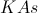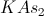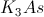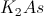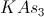Explanation:

Potassium is a Group I element, so to get to a filled valence shell, it will lost one electron, yielding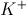.

Arsenic is a Group 5 element, so it needs to gain three electrons to obtain a filled valence shell, yielding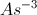.

In order to balance out the charges, the resultant salt will be.

### Example Question #1 : Balancing And Predicting Reactions

What is the net ionic equation for the ion exchange reaction between ferrous sulfate and calcium iodide? Assume all compounds are soluble.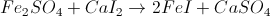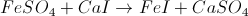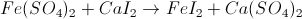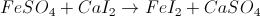Explanation:

First, we must know what ferrous sulfate is. Ferrous refers to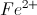, and sulfate has the formula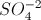. When we combine the two together we get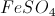.

Calcium is a divatent cation and iodide is a monovalent anion, so their salt is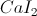. The ion exchange reaction is then:### Example Question #2 : Balancing And Predicting Reactions

Select the net ionic equation from this molecular reaction: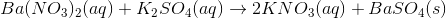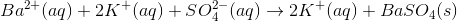None of the other choices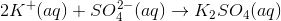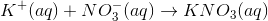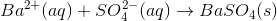Explanation:

The net ionic equation is derived by removing all spectator ions from the total ionic equation (in which all ions are listed). To put it another way, the net ionic equation involves only the ions that participate in a reaction which, in this case, is the precipitation of barium sulfate.

Begin by writing all aqueous compounds in their dissociated (ionic) forms.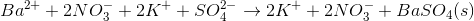Cancel out any ions that appear in equal quantities on both sides of the equation. In this case, we can cancel the nitrate and potassium ions.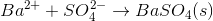This is our net ionic equation.

### Example Question #1 : Reaction Chemistry

What is the balanced chemical equation for the combustion of butane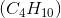?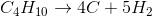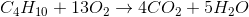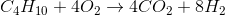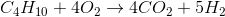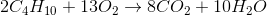Explanation:

Combustion is the chemical reaction of a hydrocarbon with molecular oxygen, and it always produces carbon dioxide and water. Knowing the reactants and products, the unbalanced equation must be: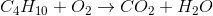We start by balancing the hydrogens. Since there are 10 on the left and only 2 on the right, we put a coefficient of 5 on water.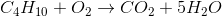Similarly, we balance carbons by putting a 4 on the carbon dioxide.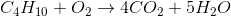To find the number of oxygens on the right, we multiply the 4 coefficient by the 2 subscript on O (which gets us 8 oxygens) and then add the 5 oxygens from the 5 water molecules to get a total of 13. The needed coefficient for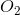on the left would then have to be 13/2.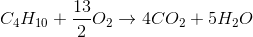Because fractional coefficients are not allowed, we mutiply every coefficient by 2 to find our final reaction: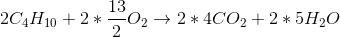### Example Question #8 : Balancing Chemical Equations

Determine whether or not solid aluminum reacts with aqueous zinc chloride. If it does, determine the balanced equation for the reaction.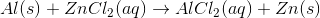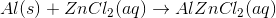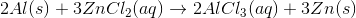No reaction occurs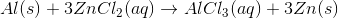Explanation:

When we check the activity series, it is fairly easy to see that aluminum metal is more reactive than zinc metal. So, in this case, the two metals undergo a redox reaction, where the aqueous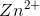is reduced to solid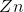, and the solid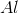is oxidized to aqueous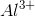. These charges are the common oxidation states for zinc and aluminum and should be memorized.

Becauseis the new species, it bonds with 3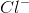ions. The unbalanced equation is: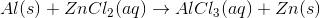We note that there are 2 chlorine atoms on the left and 3 chlorine atoms on the right. To balance, we use a 3 coefficient on the left and a 2 coefficient on the right. This gives a total of 6 chlorine atoms on eahc side.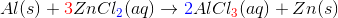However, now we have also increased the amounts of zinc and aluminum. We copy the necessary coefficients to balance those—2 for aluminum on the left, 3 for zinc on the right—and we are done:### Example Question #1 : Balancing And Predicting Reactions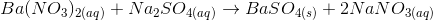What is the net ionic form of this equation?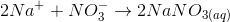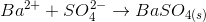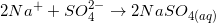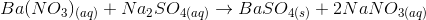Explanation:

To find the net ionic form of this chemical equation, first balance the equation, then dissociate all soluble components. In other words, separate the aqueous terms into two separate molecules/atoms. For example,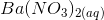dissociates into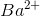and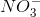. After separating all soluble terms, balance the equation and cancel out any terms that repeat on both sides of the equation. For example,will be on both sides of the equation after separating all terms so you can cross them out. Below are the steps taken to find the net ionic form of this chemical equation.Write out the dissociated forms of each species, if applicable.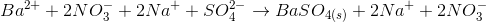Cross out species that appear on both sides of the equation. The net ionic equation is:### Example Question #2 : Balancing And Predicting Reactions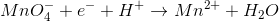What is the coefficient for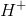, reduced to the lowest whole number, when the given reaction is balanced?The best way to solve this problem is to try all the possible answers. When we try 8,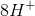means that there must be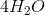means that there must be just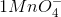, which means that there is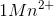. When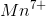is reduced to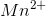, there are 5 remaining electrons. So 8 is correct. Alternatively, this question may be answered by the classical "trial and error" method of balancing equations.